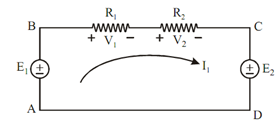## Kirchhoffs Voltage Law Assignment Help

Assignment Help: >> Kirchhoffs Laws - Kirchhoffs Voltage Law

Kirchhoff's Voltage Law (KVL):

This law is depending on the law of conservation of energy. According to this, electrical energy supplied to any circuit (closed loop) is equal to the energy consumed by its passive elements.

As this law associate the voltage in a closed circuit of an electrical network it is known as Kirchhoff's Voltage Law (KVL) or Kirchhoff's Mesh Law.

Kirchhoff's voltage law states that in a closed electric circuit the algebraic sum of emfs and voltage drops is zero.

By convention, the emfs or voltage increase are taken to be positive and voltage drops are taken to be negative.Figure: Closed Loop

In the closed circuit ABCDA, illustrated in Figure, applying Kirchhoff's voltage law, we have

E1  - V1  - V2  - E2  = 0

E1  - IR1  - IR2  - E2  = 0

E1  - E2  = IR1  + IR2

Sum of voltage rises = Sum of voltage drop or

Energy supplied = Energy consumed.